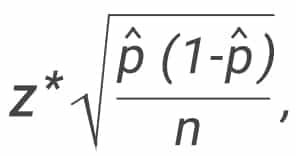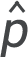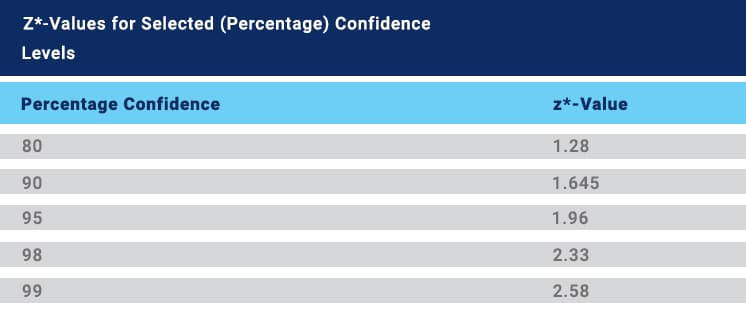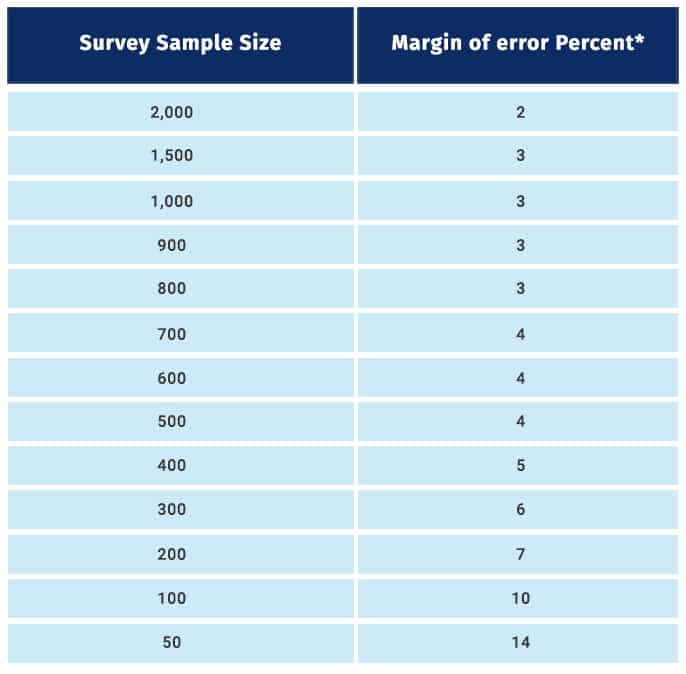# What is Margin of Error – Definition, Calculation with Examples

## Margin of Error

Definition:

Margin of errors, in statistics, is the degree of error in results received from random sampling surveys. A higher margin of error in statistics indicates less likelihood of relying on the results of a survey or poll, i.e. the confidence on the results will be lower to represent a population. It is a very vital tool in market research as it depicts confidence level the researchers should have in the data obtained from surveys.

A confidence interval is the level of unpredictability with a specific statistic. Usually, it is used in association with the margin of errors to reveal the confidence a statistician has in judging the results of an online survey or online poll are worthy to represent the entire population or not.

Lower margin of error indicates higher confidence levels in the produced results.

When we select a representative sample to estimate full population, it will have some element of uncertainty. We need to infer the real statistic from sample statistic. This means our estimate will be close to the actual figure. Considering margin of error further improves this estimate.

## Margin of Error Calculation:

A well-defined population is a prerequisite for calculating margin of error. In statistics, a “population” comprises of all the elements of a particular group that a researcher intends to study and collect data. This error can be significantly high if the population is not defined or in cases where the process of sample selection is not carried out properly.

Every time a researcher conducts a statistical survey, margin of error calculation is required. The universal formula for the margin of error for a sample iswhere:= sample proportion (“P-hat”).

n = sample size

z = z-score corresponds to your desired confidence levels.

Are you feeling a bit confused? Don’t worry! you can use our margin of error calculator.

### Example for margin of error calculation

For example, wine tasting sessions conducted in vineyards are dependent on the quality and taste of the wines presented during the session. These wines represent the entire production and depending on how well they’re received by the visitors, the feedback from them is generalized to the entire production.

The wine tasting will be effective only when visitors do not have a pattern, i.e. they’re chosen randomly. Wine goes through a process to be palatable and similarly, the visitors also must go through a process to provide effective results.

The measurement components prove whether the wine bottles are worthy to represent the entire winery’s production or not. If a statistician states that the conducted survey will have a margin of error of plus or minus 5% at a 93% confidence interval. This means that if a survey was conducted 100 times with vineyard visitors, feedback received will be within a percentage division either higher or lower than the percentage that’s accounted 93 out of 100 times.

In this case, if 60 visitors report that the wines were extremely good. As the margin of error is plus or minus 5% in a confidence interval is 93%, in 100 visitors, it’s safe to conclude that the visitors who comment that the wines were “extremely good” will be 55 or 65 (93%) of the time.

To explain this further, let’s take an example of a survey on volunteering was sent to 1000 respondents out of which 500 agreed to the statement in the survey saying that volunteering makes life better. Calculate margin of error for 95% confidence level.

Step 1: Calculate P-hat by dividing the number of respondents who agreed with the statement in the survey to the total number of respondents. In this case, = 500/1000 = 50%

Step 2: Find z-score corresponding to 95% confidence level. In this case, z score is 1.96

Step 3: Calculate by putting these values in the formula

Step 4: Convert to a percentage### Margin of error in sample sizes:

In probability sampling, each member of a population has a probability of being selected to be a part of the sample. In this method, researchers and statisticians can select members from their area of research so that the margin of error in data received from these samples is as minimum as possible.

In non-probability sampling, samples are formed on the basis of cost-effectiveness or convenience and not on the basis of application and because of this selection process, some sections of the population may get excluded. Surveys will be effective only on filtering members according to interests and application to the survey being conducted.

The industrial standard for confidence level is 95% and these are the margin of error percentages for certain survey sample sizes:As indicated in this table, to reduce the margin of error to half, for instance from 4 to 2, the sample size has been increased considerably, from 500 to 2000. As you must have observed, the sample size is inversely proportional to the margin of error. Till sample sizes of 1500, there is a significant decrease in margin of error but beyond that, this decrease reduces.